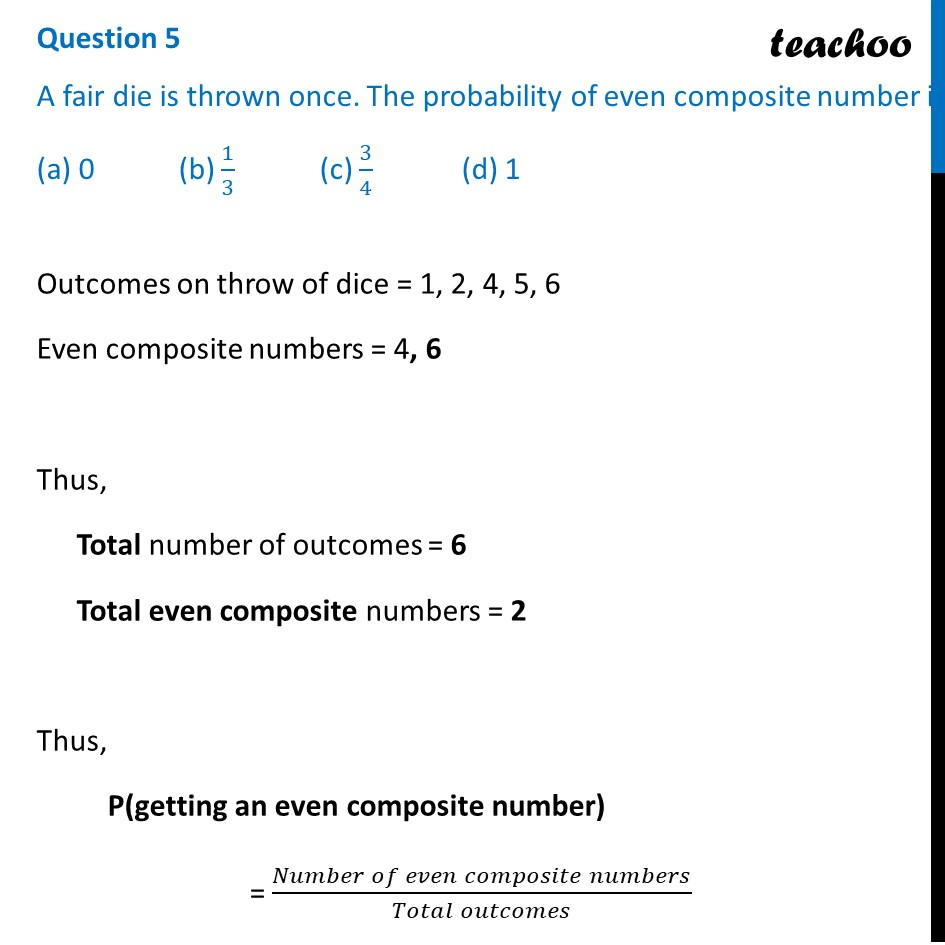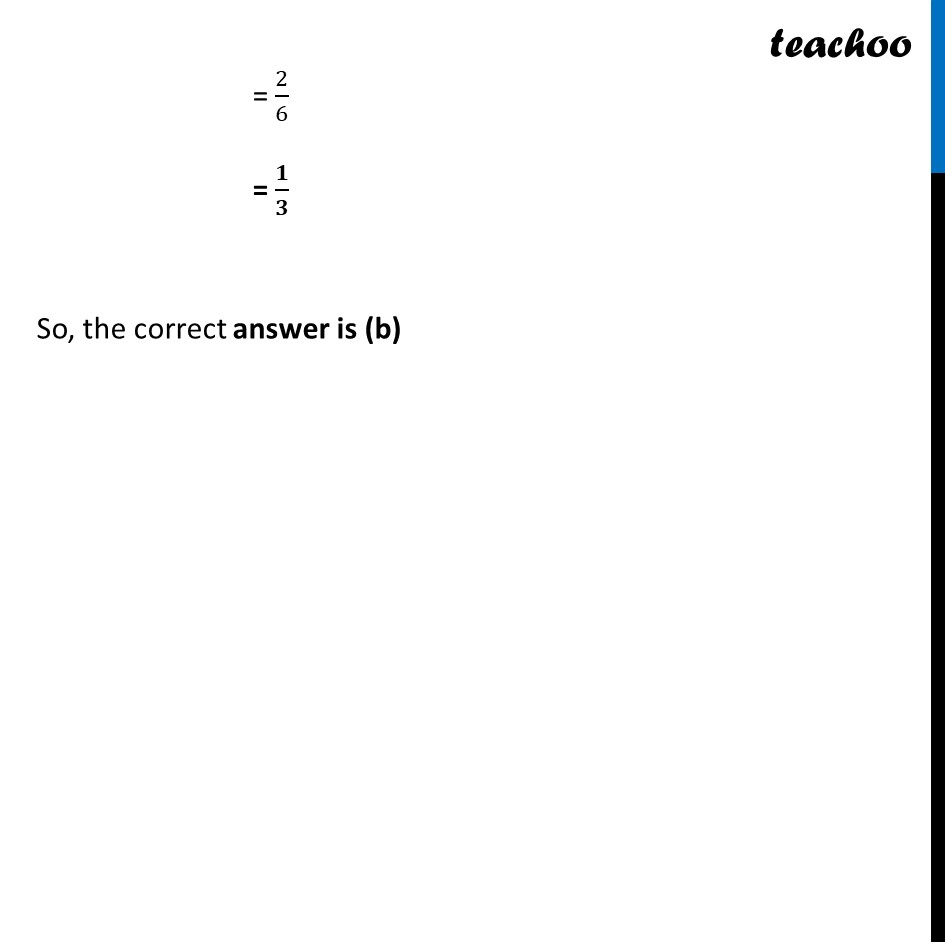## (a) 0   (b) 1/3  (c) 3/4  (d) 11. Class 10
2. Solutions of Sample Papers for Class 10 Boards
3. CBSE Class 10 Sample Paper for 2022 Boards - Maths Basic [MCQ]

Transcript

Question 5 A fair die is thrown once. The probability of even composite number is (a) 0 (b) 1/3 (c) 3/4 (d) 1 Outcomes on throw of dice = 1, 2, 4, 5, 6 Even composite numbers = 4, 6 Thus, Total number of outcomes = 6 Total even composite numbers = 2 Thus, P(getting an even composite number) = (𝑁𝑢𝑚𝑏𝑒𝑟 𝑜𝑓 𝑒𝑣𝑒𝑛 𝑐𝑜𝑚𝑝𝑜𝑠𝑖𝑡𝑒 𝑛𝑢𝑚𝑏𝑒𝑟𝑠)/(𝑇𝑜𝑡𝑎𝑙 𝑜𝑢𝑡𝑐𝑜𝑚𝑒𝑠) = 2/6 = 𝟏/𝟑 So, the correct answer is (b)